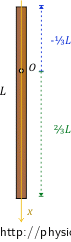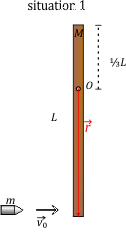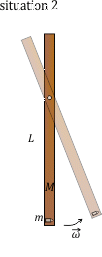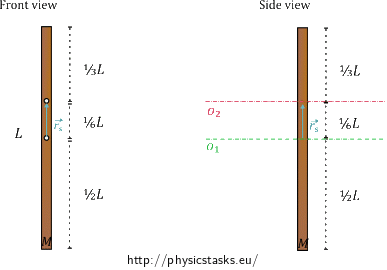## A Bullet and a Rod II

A bullet of mass $$m$$ travelling horizontally with velocity $$\vec{v_{0}}$$ hits the end of a wooden rod of length $$L$$ and mass $$M$$ and gets stuck in it. The rod is free to rotate around a horizontal axis passing through the rod at $$\frac{1}{3}$$ of its length (at a distance of $$\frac{2}{3}L$$ from the end where it is hit).

a) Determine the moment of inertia of the rod with respect to the axis of rotation.

b) Calculate the angular velocity with which the rod starts to rotate.

c) Calculate the ratio of the change in internal energy of the system of the bullet and the rod $$\Delta U$$ to the initial kinetic energy of this system $$E_{k_{0}}$$.

• #### Analysis

We will assume in this task that the bullet penetrates the rod very quickly, stops inside it, and then the rod starts to rotate with the angular velocity we aim to determine.

We will use the law of conservation of angular momentum to solve the task. We will focus on two situations; the first is just before the moment when the bullet hits the rod, and the second is the moment just after the hit. We will write down the total angular momentum of the system of the bullet and the rod in both of these situations and we will express the angular velocity we aim to determine from the equality of the magnitudes of the angular momenta.

When the bullet penetrates the rod, both the bullet and the rod heat up and deform, the mechanical energy is thus not conserved. We will calculate the change in internal energy from the difference of the total mechanical energy of the system of the bullet and the rod before the hit and after it.

• #### Hint for a)

To determine the moment of inertia of the rod $$I_{rod}$$, we start with the following equation:

$I_{rod} = \int_V{\rho r^2}\,\mathrm{d}V,$

where r is the distance of a segment of volume $$\mathrm{d}V$$ from the axis of rotation and $$\rho$$ is the density of the rod.

Think about the possible ways to simplify the integral when we assume that the rod is homogenous and thin. What limits of integration would the integral have if the axis was passing through the end of the rod? How do the limits of integration change when the axis is passing through the rod at $$\frac{1}{3}$$ of its length?

• #### Hint for b)

Let us assume that the bullet penetrates the rod very quickly, stops in it, then the bullet and the rod start moving together. To solve question b), we use one of the laws of conservation. Which physical quantity is conserved in this situation?

• #### Hint for c)

Write down the expression of mechanical energy of the system of the bullet and the rod before the bullet hits the rod and just after the hit. Think about the magnitudes of the energies; are they the same or not? How do you express the change in the internal energy of the system?

• #### Solution of a)

We calculate the moment of inertia I from the following integral:

$I_{rod} = \int_V{\rho r^2}\,\mathrm{d}V,$

where r denotes the distance of a segment of volume $$\mathrm{d}V$$ from the axis of rotation and $$\rho$$ is the density of the rod. We assume the rod to be infinitely thin. It suffices, therefore, to calculate the integral in one dimension only. We rewrite the integral:

$I_{rod} = \int{\lambda r^2}\,\mathrm{d}r, \tag{1}$

where $$\lambda$$ is the linear density of the rod (which characterizes the distribution of the total mass $$M$$ of the rod along its length $$L$$). Because we consider the rod to be homogenous, it holds for the linear density $$\lambda$$:

$\lambda = \frac{M}{L} . \tag{2}$

We know that the axis of rotation passes through the rod at $$\frac{1}{3}$$ of its length. We, therefore, integrate from $$−\frac{L}{3}$$ to $$\frac{2}{3}L$$ when calculating the moment of inertia to include the entire length of the rod.Substituting expression (2) in integral (1) and writing down the integration limits, we get for the moment of inertia of the rod $$I_{rod}$$:

$I_{rod} = \int_{−\frac{L}{3}}^{\frac{2}{3}L}{\frac{M}{L} r^2}\,\mathrm{d}r =\frac{M}{L} \int_{−\frac{L}{3}}^{\frac{2}{3}L}{ r^2}\,\mathrm{d}r = \frac{M}{L} \left[\frac{r^3}{3}\right]_{−\frac{L}{3}}^{\frac{2}{3}L} = \frac{M}{L}\left[\frac{8}{27{\cdot} 3}L^3−\left(−\frac{1}{27{\cdot} 3}L^3\right)\right] =ML^2 \left(\frac{9}{27 {\cdot} 3}\right).$

Simplifying the fraction, we get:

$I_{rod} = \frac{1}{9}ML^2.$

Note: For a more detailed derivation of the integral, see the task A Bullet and a Rod I.

• #### Solution of b)

We will use the law of conservation of angular momentum to determine the angular velocity $$\vec \omega$$ with which the rod starts to rotate. We will compare two situations when the total angular momentum of the system of the bullet and the rod must be equal.An angular momentum $$\vec{L}$$ of a mass point with respect to a point O is defined as the following cross product:

$\vec L = \vec {r}×\vec{p},$

where $$\vec{r}$$ is the position vector (in our case pointing from O to the end of the rod where the bullet got stuck), $$\vec p$$ is the momentum of the mass point.

It holds for a magnitude of the cross product:

$L = rp\sin\varphi \,,$

where φ is the angle between the vectors.

The vector $$\vec{L}$$ of angular momentum is perpendicular to a plane defined by vectors $$\vec{r}$$ and $$\vec{p}$$. We determine the orientation of the angular momentum using the right-hand rule: we curl the fingers to represent a motion from $$\vec{r}$$ to $$\vec{p}$$, the thumb then points along the direction of the vector $$\vec{L}$$. The vector $$\vec p$$ points in the same direction as the vector of velocity $$\vec {v_0}$$.

It holds for an angular momentum $$\vec{L}$$ of a rigid body with respect to a fixed axis of rotation that:

$\vec{L} = I\vec{\omega}\,,$

where I is the moment of inertia of the body with respect to the fixed axis of rotation and $$\vec{\omega}$$ is the angular velocity with which the body rotates.

For the magnitude of angular momentum, we have:

$L = I\omega\,.$

The orientation of vector $$\vec{L}$$ of angular momentum is the same as the orientation of the vector $$\vec{\omega}$$ of angular velocity.

We will determine the angular momentum of the system of the bullet and the rod with respect to point O where the rod is attached.

In the beginning, the rod is at rest. The total angular momentum of the system is thus equal to the angular momentum of the bullet, which is moving at the speed $$\vec {v_0}$$ in a direction perpendicular to the rod. Angular momentum for the first situation is:

$\vec {L_{1}} = \vec {r}×\vec{p}= \vec {r}×\left (m\vec{v_{0}}\right ).$

The magnitude of the radius vector $$\vec{r}$$ equals $$\frac{2}{3}$$ of the length of the rod L. From knowing that sine of the right angle defined by the momentum $$\vec p$$ and the radius vector $$\vec r$$ is 1, we get for the magnitude of the angular momentum:

$L_{1} = \frac{2}{3}Lp = \frac{2}{3}mv_{0}L . \tag{3}$

In the second situation, we also have to consider the rotational motion of the rod around the axis. We determine the angular momentum $$\vec L_{2}$$ as a product of the total moment of inertia of the system $$I$$ and its angular velocity $$\vec{\omega}$$. The total moment of inertia $$I$$ is composed of the moment of inertia of the rod Irod (we determined it in the previous part of the task) and the moment of inertia of the bullet Ibullet, which we assume to be a mass point in the distance of $$\frac{2}{3}L$$ from the axis of rotation. Because the rod and the bullet are moving with the same angular velocity, we can simply sum their moments of inertia up and get the total moment of inertia $$I$$.

$I = I_{rod} + I_{bullet} = \frac{1}{9}ML^2 + m\left(\frac{2}{3}L\right)^2 \tag{4}$

It thus holds for the angular momentum $$\vec L_{2}$$:

$\vec{L_{2}} = I\vec{\omega} = \left(\frac{1}{9}ML^2 + m\left(\frac{2}{3}L\right)^2\right)\vec{\omega}$

and for its magnitude:

$L_{2} = I\omega = \left(\frac{1}{9}ML^2 + m\left(\frac{2}{3}L\right)^2\right){\omega}. \tag{5}$

Equality of relations (3) and (5) follows from the law of conservation of angular momentum:

$L_1=L_2$

$\frac{2}{3}mv_{0}L = \left(\frac{1}{9}ML^2 + m\frac{4}{9}L^2\right)\omega$

We express the angular velocity $$\omega$$:

$\frac{2}{3}mv_{0} = \left(\frac{1}{9}M+ m\frac{4}{9}\right)L\omega,$

$2mv_{0} = \left(\frac{1}{3}M+ m\frac{4}{3}\right)L\omega,$

$\omega = \frac{2mv_{0}}{\left(\frac{1}{3}M + \frac{4}{3}m\right)L}.$

Rearranging the expression, we get:

$\omega = \frac{6mv_{0}}{\left(M + 4m\right)L}.$

• #### Solution of c)

The solution process is the same as in the last section of the task A Bullet and a Rod I. You can find a detailed explanation there.

We want to determine the ratio of the change in internal energy of the system of the bullet and the rod to the initial kinetic energy of this system. The initial kinetic energy of the system is the same as the initial kinetic energy of the bullet:

$E_{k_{0}} = \frac{1}{2}mv_{0}^2.$

We get the change in the internal energy $$\Delta U$$ as a difference of the initial kinetic energy $$E_{k_{0}}$$ and the energy of the rotational movement $$E_k$$, for which it holds:

$E_k = \frac{1}{2}I\omega^2.$

We determined the total moment of inertia $$I$$ of the system of the bullet and the rod in the previous part of the task; it is the equation (4).

We know everything we need to determine the ratio we are looking for:

$\frac{\Delta U}{E_{k_{0}}} =\frac{E_{k_{0}} − E_k}{E_{k_{0}}} =\frac{\frac{1}{2}mv_{0}^2−\frac{1}{2}\left(\frac{1}{9}ML^2+\frac{4}{9}mL^2\right) \omega^2}{\frac{1}{2}mv_{0}^2}.$

We substitute from the previous part of the task for the angular velocity $$\omega$$ and rearrange the expression:

$\frac{\Delta U}{E_{k_{0}}}=\frac{\frac{1}{2}mv_{0}^2−\frac{1}{18}L^2\left(M+4m\right) \frac{36m^2v_{0}^2}{\left(M + 4m\right)^2L^2}}{\frac{1}{2}mv_{0}^2} = \frac{\frac{1}{2}m−\frac{2m^2}{\left(M + 4m\right)}}{\frac{1}{2}m} = 1−\frac{4m}{\left(M + 4m\right)}.$

We get the ratio:

$\frac{\Delta U}{E_{k_{0}}}= \frac{M}{\left(M + 4m\right)}.$

The moment of inertia of the rod with respect to the horizontal axis of rotation passing through the rod in one third of its length is:

$I_{rod} = \frac{1}{9}ML^2.$

The angular velocity with which the system of the bullet and rod starts to rotate is:

$\omega = \frac{6mv_{0}}{\left(M + 4m\right)L}.$

The ratio of the change in internal energy of the system of the bullet and the rod $$\Delta U$$ to the initial kinetic energy of this system $$E_{k_{0}}$$ is:

$\frac{\Delta U}{E_{k_{0}}}= \frac{M}{\left(M + 4m\right)}.$

• #### A different way to derive moment of inertia

We can find the moment of inertia of the rod in a different way than shown in the first part of this task. That is by using the parallel axis theorem.

We start with the relation for the moment of inertia of the rod with respect to the axis passing through the centre of mass of the rod:

$I_{1} = \frac{1}{12}ML^2.$

The parallel axis theorem states that: We determine the moment of inertia of the body with respect to the axis parallel to the axis passing through the centre of mass of the body as a sum of the moment of inertia about the axis passing through its centre of mass (i.e. $$I_{1}$$) and the square of the position vector $$\vec{r_{s}}$$ which determines the distance of the two axes times the mass of the body:

$I_{2} = I_{1} + M\left(\vec {r_{s}}\right)^2.$

In our case, the distance of the two axes $$\left|\vec{r_{s}}\right|$$ is equal to $$\frac{1}{6}L$$ (see the figure below).We get:

$I_{2} = \frac{1}{12}ML^2 + M\left(\frac{1}{6}L\right)^2 = \frac{1}{12}ML^2+\frac{1}{36}ML^2 = \left(\frac{3+1}{36}\right)ML^2 = \frac{1}{9}ML^2,$

which is the same result as when we were using the integral in the solution of a).